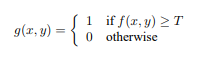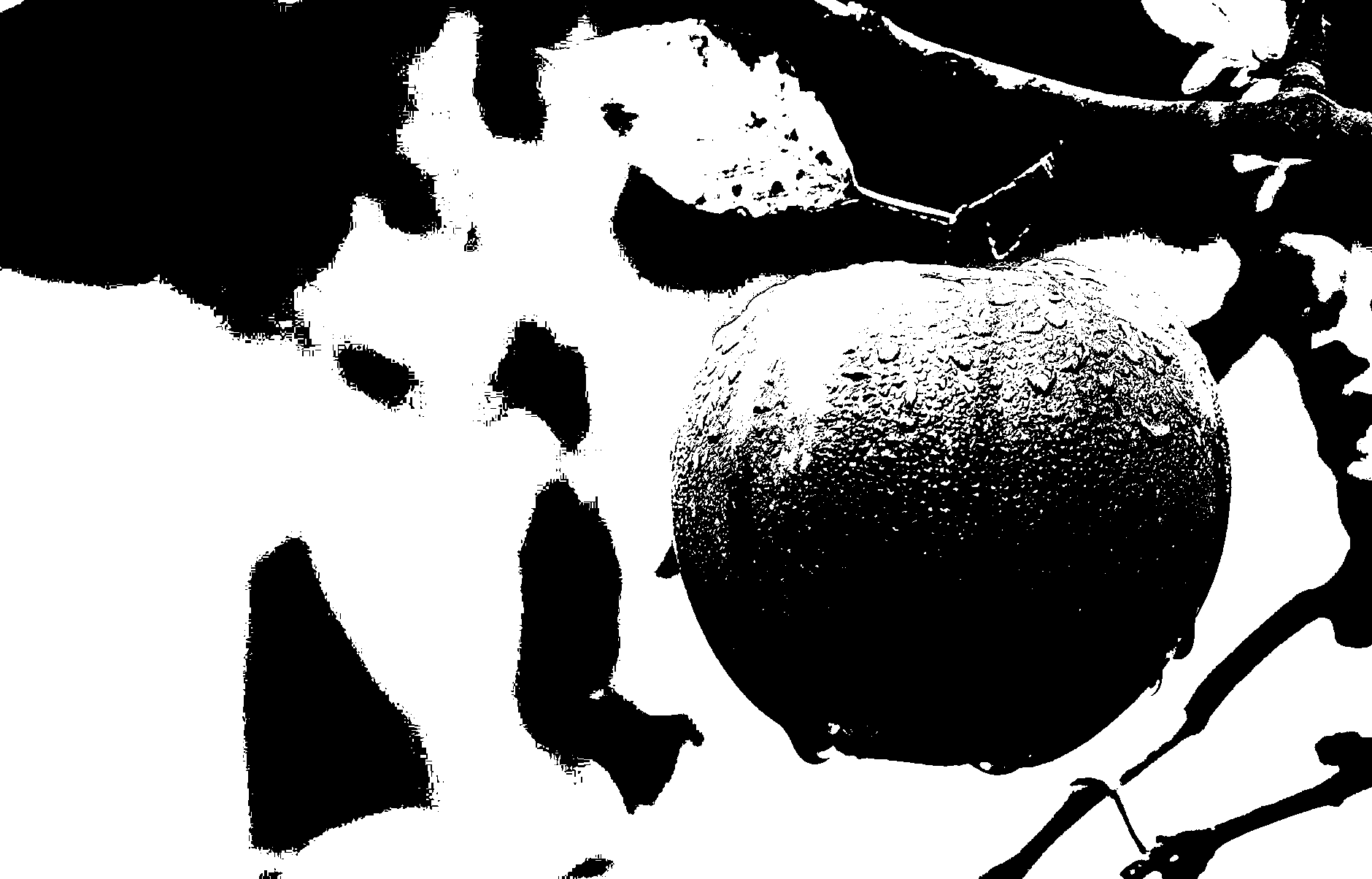# MATLAB | Converting a Grayscale Image to Binary Image using Thresholding

Thresholding is the simplest method of image segmentation and the most common way to convert a grayscale image to a binary image.

In thresholding, we select a threshold value and then all the gray level value which is below the selected threshold value is classified as 0(black i.e background ) and all the gray level which is equal to or greater than the threshold value are classified as 1(white i.e foreground).Here g(x, y) represents threshold image pixel at (x, y) and f(x, y) represents greyscale image pixel at (x, y).

Algorithm:

1. Read target image into MATLAB environment.
2. Convert it to a grayscale Image if read image is an RGB Image.
3. Calculate a threshold value, T
4. Create a new Image Array (say ‘binary’) with the same number of rows and columns as original image array, containing all elements as 0 (zero).
5. Assign 1 to binary(i, j), if gray level pixel at (i, j) is greater than or equal to the threshold value, T ; else assign 0 to binary(i, j).
Do the same for all gray level pixels.

Below is the implementation of above algorithm :

 `% Following MATLAB function will take a grayscale  ` `% or an RGB image as input and will return a ` `% binary image as output  ` `  `  `function` `[binary] = convert2binary(img)  ` `  `  `     ``[x, y, z]=size(img); ` `  `  `     ``% if Read Image is an RGB Image then convert  ` `     ``% it to a Gray Scale Image For an RGB image  ` `     ``% the value of z will be 3 and for a Grayscale ` `     ``% Image the value of z will be 1 ` `  `  `    ``if` `z==3 ` `         ``img=rgb2gray(img); ` `    ``end` `  `  `    ``% change the class of image  ` `    ``% array from 'unit8' to 'double' ` `    ``img=double(img); ` `  `  `    ``% Calculate sum of all the gray level ` `    ``% pixel's value of the GraySacle Image ` `    ``sum=0; ` `    ``for` `i=1:x ` `         ``for` `j=1:y ` `        ``sum=sum+img(i, j); ` `     ``end` `     ``end` `  `  `    ``% Calculate Threshold value by dividing the  ` `    ``% calculated sum by total number of pixels  ` `    ``% total number of pixels = rows*columns (i.e x*y)  ` `    ``threshold=sum/(x*y); ` `   `  `    ``% Create a image array having same number ` `    ``% of rows and column as Original image ` `    ``% with all elements as 0 (Zero). ` `    ``binary=zeros(x, y); ` `  `  `    ``% iterate over all the pixels of Grayscale  ` `    ``% Image and Assign 1 to binary(i, j), if gray ` `    ``% level value is >=  threshold value      ` `    ``% else assign 0 to binary(i, j) . ` `  `  `    ``for` `i=1:x ` `     ``for` `j=1:y ` `        ``if` `img(i, j) >= threshold ` `                ``binary(i, j) = 1; ` `        ``else` `            ``binary(i, j)=0; ` `        ``end` `     ``end` `    ``end` `end` `  `  `  `  `% driver function  ` `  `  `% Read the target Image ` `img=imread(``'apple.png'``); ` `  `  `% Call convert2binary() function to convert ` `% Image to binary using thresholding ` `binary_image=convert2binary(img); ` `  `  `% Display result ` `imshow(binary_image); `

Input:Output:• This method is easy to understand and simple to implement.
• It Converts a grayscale image to binary image.
• Resultant binary image is easy to analyze.

• We only consider the intensity of the image for thresholding process, but not consider any relationship between the pixels. So, the pixels identified by thresholding process might not be continuous.
• While thresholding process we can easily include the extraneous pixels that aren’t part of the desired region and can easily miss the pixels that are part of desired region.
• It is also very sensitive to noise in the image. The result of thresholding process gets worse as noise gets worse.

My Personal Notes arrow_drop_upCheck out this Author's contributed articles.

If you like GeeksforGeeks and would like to contribute, you can also write an article using contribute.geeksforgeeks.org or mail your article to contribute@geeksforgeeks.org. See your article appearing on the GeeksforGeeks main page and help other Geeks.

Please Improve this article if you find anything incorrect by clicking on the "Improve Article" button below.

Article Tags :

Be the First to upvote.

Please write to us at contribute@geeksforgeeks.org to report any issue with the above content.